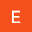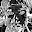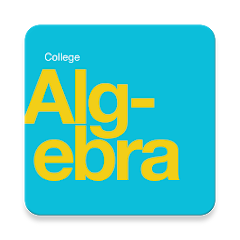# College Algebra Textbook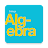4.1
27 reviews
10K+Everyone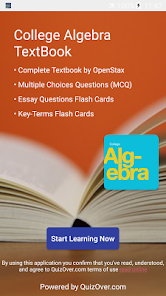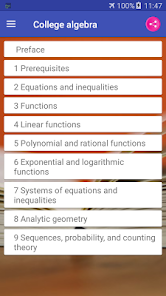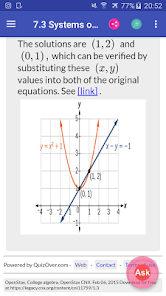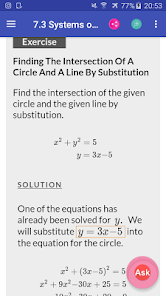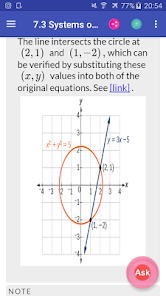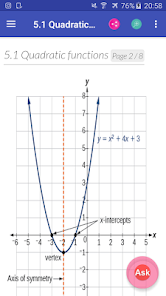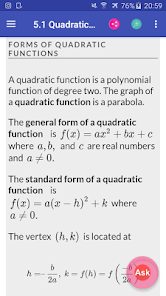College Algebra TextBook by OpenStax plus MCQ, Essay Questions & Key Terms

College Algebra provides a comprehensive exploration of algebraic principles and meets scope and sequence requirements for a typical introductory algebra course. The modular approach and richness of content ensure that the book meets the needs of a variety of courses. College Algebra offers a wealth of examples with detailed, conceptual explanations, building a strong foundation in the material before asking students to apply what they’ve learned.

* Complete Textbook by OpenStax
* Multiple Choices Questions (MCQ)
* Essay Questions Flash Cards
* Key-Terms Flash Cards

1. Prerequisites
Introduction to Prerequisites
1.1. Real Numbers: Algebra Essentials
1.2. Exponents and Scientific Notation
1.4. Polynomials
1.5. Factoring Polynomials
1.6. Rational Expressions
2. Equations and Inequalities
Introduction to Equations and Inequalities
2.1. The Rectangular Coordinate Systems and Graphs
2.2. Linear Equations in One Variable
2.3. Models and Applications
2.4. Complex Numbers
2.6. Other Types of Equations
2.7. Linear Inequalities and Absolute Value Inequalities
3. Functions
Introduction to Functions
3.1. Functions and Function Notation
3.2. Domain and Range
3.3. Rates of Change and Behavior of Graphs
3.4. Composition of Functions
3.5. Transformation of Functions
3.6. Absolute Value Functions
3.7. Inverse Functions
4. Linear Functions
Introduction to Linear Functions
4.1. Linear Functions
4.2. Modeling with Linear Functions
4.3. Fitting Linear Models to Data
5. Polynomial and Rational Functions
Introduction to Polynomial and Rational Functions
5.2. Power Functions and Polynomial Functions
5.3. Graphs of Polynomial Functions
5.4. Dividing Polynomials
5.5. Zeros of Polynomial Functions
5.6. Rational Functions
5.8. Modeling Using Variation
6. Exponential and Logarithmic Functions
Introduction to Exponential and Logarithmic Functions
6.1. Exponential Functions
6.2. Graphs of Exponential Functions
6.3. Logarithmic Functions
6.4. Graphs of Logarithmic Functions
6.5. Logarithmic Properties
6.6. Exponential and Logarithmic Equations
6.7. Exponential and Logarithmic Models
6.8. Fitting Exponential Models to Data
7. Systems of Equations and Inequalities
Introduction to Systems of Equations and Inequalities
7.1. Systems of Linear Equations: Two Variables
7.2. Systems of Linear Equations: Three Variables
7.3. Systems of Nonlinear Equations and Inequalities: Two Variables
7.4. Partial Fractions
7.5. Matrices and Matrix Operations
7.6. Solving Systems with Gaussian Elimination
7.7. Solving Systems with Inverses
7.8. Solving Systems with Cramer's Rule
8. Analytic Geometry
Introduction to Analytic Geometry
8.1. The Ellipse
8.2. The Hyperbola
8.3. The Parabola
8.4. Rotation of Axes
8.5. Conic Sections in Polar Coordinates
9. Sequences, Probability, and Counting Theory
Introduction to Sequences, Probability and Counting Theory
9.1. Sequences and Their Notations
9.2. Arithmetic Sequences
9.3. Geometric Sequences
9.4. Series and Their Notations
9.5. Counting Principles
9.6. Binomial Theorem
9.7. Probability
Updated on
Mar 20, 2018

## Data safety

Safety starts with understanding how developers collect and share your data. Data privacy and security practices may vary based on your use, region, and age. The developer provided this information and may update it over time.No data shared with third partiesNo data collected

## Ratings and reviews

4.1
25 reviews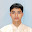John Myre Napoles
June 22, 2020
Great job! This app is amazing it helps me a lot to improve my mathematical skills. As my experience i haven't spend money for this app but they wanted to help students. Thank you very much for this application i really appreciate it. Keep it up❤
6 people found this review helpful